## Data Structures and Algorithms 2, Fall 1998 Problem Set 2 (16-18 Nov.)

1. Show that if the selection of the pivot element v in the Quicksort algorithm is done as indicated on p. 31 of the lecture notes (i.e. choose the larger one of the two first distinct values found), then the Quicksort algorithm requires time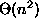in the worst case. Give an example of an input sequence that leads to this quadratic behaviour.
2. Verify, by induction, that any solution to the following recurrence (arising from the analysis of the linear-time median algorithm):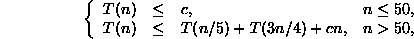satisfies the condition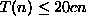for all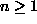.

3. Suppose we were to come up with a variant of Strassen's matrix multiplication algorithm based on the fact that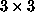matrices can be multiplied in only m multiplications instead of the normal 27. How small would m have to be for this algorithm to be faster than Strassen's algorithm for large enough n?
4. V. Pan has discovered a way of multiplying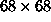matrices using 132464 multiplications, a way of multiplying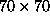matrices using 143640 multiplications, and a way of multiplying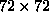matrices using 155424 multiplications. Which method yields the best asymptotic running time when used in a divide-and-conquer matrix multiplication algorithm? Compare it with the running time of Strassen's algorithm.
5. Implement, on your favorite computer system, one of the following three algorithms: (a) the Karatsuba-Ofman algorithm for integer multiplication, (b) Strassen's matrix multiplication algorithm, (c) the deterministic linear-time median algorithm. Compare the running time of your program to some standard method for the same problem. How large must the input values be before the asymptotically faster algorithms supercedes the standard method?

Hint for case (a): one ``standard'' method for long-integer multiplication is the following algorithm:

```
multiply(x,y);

{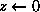;

y > 0

{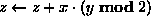;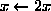;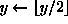};

(z)

}

```

Pekka Orponen
Thu Nov 12 12:49:09 EET 1998